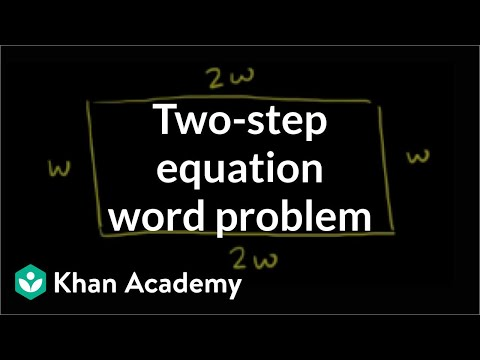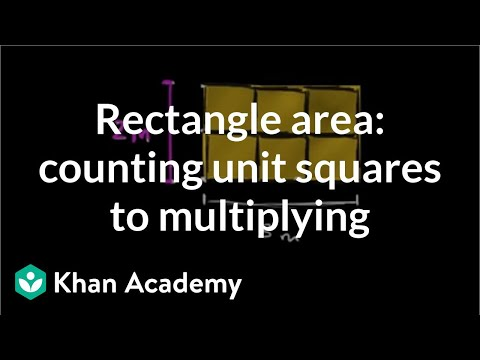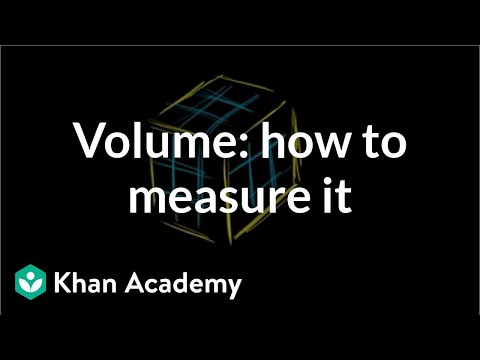# Defined and undefined matrix operations

##By Khan Academy

Sal discusses the conditions of matrix dimensions for which addition or multiplication are defined.# Multiplying a matrix by a matrix

##By Khan Academy

Sal gives an example of a multiplication of two matrices that don't have the same dimensions.# Defined and undefined matrix operations

##By Khan Academy

Sal gives an example of a multiplication of two matrices that don't have the same dimensions.# Example Problem - Multiplying Matrices with Different Dimensions

##By Khan Academy

The instructor uses an electronic chalkboard to demonstrate Matrix Multiplication. At the time of this posting from WatchKnow, this video was a Featured Video on YouTube. The instructor, Sal Khan, continues from Part 1 on multiplying matrices. The student or educator may want to open the video to 'full screen' as the instructor is using a black background and the writing is small.# Linear equation word problems

##By Khan Academy

Here's a nifty word problem in which we find the dimensions of a garden given only the perimeter. Let's create an equation to solve!# Linear equation word problems

##By Khan Academy

Here's a nifty word problem in which we find the dimensions of a garden given only the perimeter. Let's create an equation to solve!# Linear equation word problems

##By Khan Academy

Here's a nifty word problem in which we find the dimensions of a garden given only the perimeter. Let's create an equation to solve!# Rectangle area as product of dimensions same as counting unit squares | Pre-Algebra

##By Khan Academy

Use unit squares to see why multiplying side-lengths can also find the area of rectangles. ï¿½ï¿½ï¿½ï¿½ï¿½ï¿½ï¿½ï¿½ï¿½ï¿½ï¿½ï¿½ï¿½ï¿½ï¿½ï¿½ï¿½ï¿½ï¿½ï¿½ï¿½ï¿½ï¿½ï¿½# Rectangle area as product of dimensions same as counting unit squares

##By Khan Academy

Use unit squares to see why multiplying side-lengths can also find the area of rectangles. ï¿½ï¿½ï¿½ï¿½ï¿½ï¿½ï¿½ï¿½ï¿½ï¿½ï¿½ï¿½ï¿½ï¿½ï¿½ï¿½ï¿½ï¿½ï¿½ï¿½ï¿½ï¿½ï¿½ï¿½# Volume with unit cubes 1

##By Khan Academy

Volume is a measurement in the 3rd dimension. This means that it not only has width and height, but it has depth as well. Let's watch this video in which we get a great explanation of how we measure volume.# Volume with unit cubes 1

##By Khan Academy

Volume is a measurement in the 3rd dimension. This means that it not only has width and height, but it has depth as well. Let's watch this video in which we get a great explanation of how we measure volume.# Volume with fractions

##By Khan Academy

Learn how to find the volume of a rectangular prism that has fractional side lengths. The prism in this video has dimensions 3/5 by 1 1/6 by 3/7.# Volume with unit cubes 1

##By Khan Academy

Volume is a measurement in the 3rd dimension. This means that it not only has width and height, but it has depth as well. Let's watch this video in which we get a great explanation of how we measure volume.# Volume with unit cubes 1

##By Khan Academy

Volume is a measurement in the 3rd dimension. This means that it not only has width and height, but it has depth as well. Let's watch this video in which we get a great explanation of how we measure volume.# Volume with unit cubes 1

##By Khan Academy

Volume is a measurement in the 3rd dimension. This means that it not only has width and height, but it has depth as well. Let's watch this video in which we get a great explanation of how we measure volume.# Volume with unit cubes 1

##By Khan Academy

Volume is a measurement in the 3rd dimension. This means that it not only has width and height, but it has depth as well. Let's watch this video in which we get a great explanation of how we measure volume.# Volume with unit cubes 1

##By Khan Academy

Volume is a measurement in the 3rd dimension. This means that it not only has width and height, but it has depth as well. Let's watch this video in which we get a great explanation of how we measure volume.# Volume with unit cubes 1

##By Khan Academy

Volume is a measurement in the 3rd dimension. This means that it not only has width and height, but it has depth as well. Let's watch this video in which we get a great explanation of how we measure volume.# Quadratic Model: Enclosing a field with a fence

##By SuperMathPrincess

How to create a quadratic function that represents the area of a rectangular field as a function of the width of the field, given a specific amount of fencing to enclose the field. Also includes how to find the maximum area and the dimensions of the field needed to obtain that maximum area, both by hand and by using a TI-83 plus graphing calculator.# Introduction to Conversions in Physics

##By Flipping Physics

This video includes the basics of doing conversions, why conversions work, conversions with squares and fractions. Includes several examples Times of the examples: 0:59 - 11 millimeters to meters 2:54 - 4.2 cm to m 3:35 - 17 g to kg 4:32 - 14 m/s to km/hr 7:40 - 12.2 mm^2 to m^2 9:50 - 120 km/hr^2 to m/s^2 Want Lecture Notes? Next Video: Introduction to Accuracy and Precision (includes Relative Error) Previous Video: Introduction to Base Dimensions and Your Friends